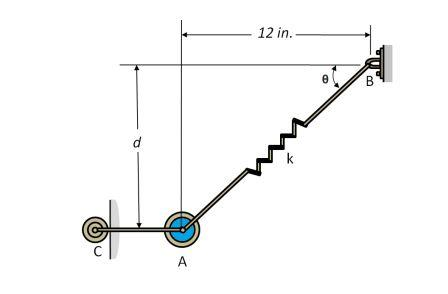# The 10-lb weight ''A'' is supported by the cord ''AC'' and roller ''C''. and by spring ''AB''. If...

## Question:

The 10-lb weight A is supported by the cord AC and roller C. and by spring AB. If the spring has an unstretched length of 8 in. and the weight is in equilibrium when d = 4 in. Determining the stiffness k of the spring.## Hooke's Law:

The spring extends on applying the tensile force and gets compressed due to the compressive force. The force responsible for the deformation of the spring is linearly proportional to the stretch or compression in the spring. Mathematically:

{eq}\rm F=kx {/eq}

where:

• {eq}k {/eq} is the stiffness of the spring
• {eq}x {/eq} is the stretch or compression in the spring

## Answer and Explanation: 1

Become a Study.com member to unlock this answer! Create your account

Given data:

• {eq}W=\rm 10 \ lb {/eq} is the weight attached at A
• {eq}F_{AB} {/eq} is the spring force
• {eq}k {/eq} is the spring constant
• {eq}x {...

See full answer below.

#### Learn more about this topic:Hooke's Law & the Spring Constant: Definition & Equation

from

Chapter 4 / Lesson 19
202K

After watching this video, you will be able to explain what Hooke's Law is and use the equation for Hooke's Law to solve problems. A short quiz will follow.